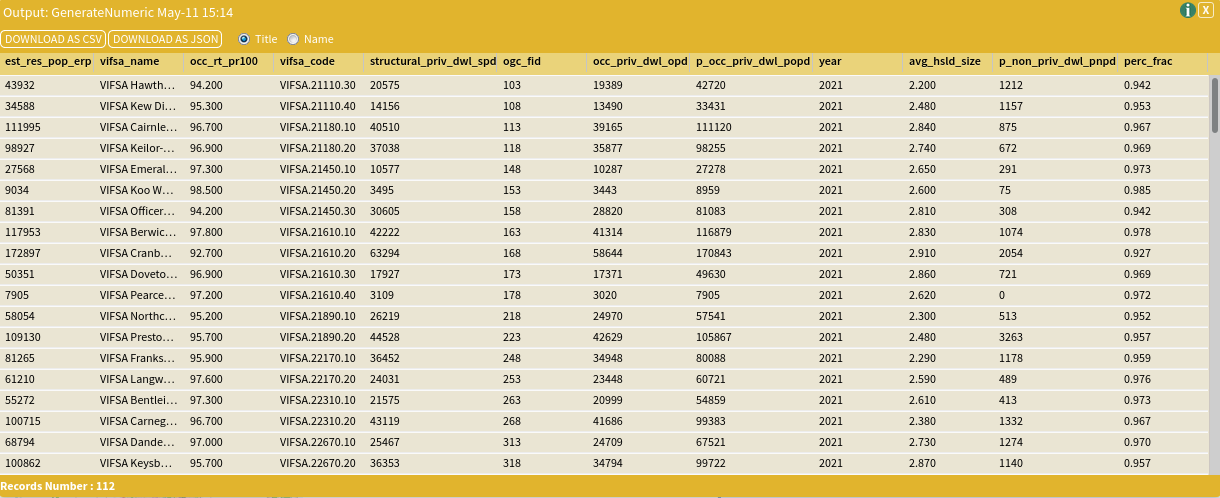Generate Numeric

The Generate Numeric tool allows you to create new columns and variables that are the result of manipulating one column by a simple mathematical function.

SET UP

In this tutorial, we will calculate the value of a percentage given in our dataset from between the ranges of 0 and 100, to that of a fraction between 0 and 1. We are doing this as all of the other datasets we have imported represent percentages this way and will simplify our analysis later. We will do this to a dataset on Household Dwellings supplied by DELWP Victoria.

To do this:

• Select Greater Melbourne (gccsa_2016/2GMEL) as your area
• Select VIC DELWP – Victoria in Future 2016 – ERP by Household Dwellings (VIFSA) 2011-2031 as your dataset, selecting all attributes, and with the following attribute filter:
• Year: 2021

Once you have completed these steps, you are ready to use the Generate Numeric tool – follow the inputs instructions below to see how to do this.

Inputs

Once you have performed the generate function, open the Generate Numeric tool (Tools → Data Processing → Generate Numeric) and enter the parameters as shown in the image below (each of the parameters is explained in more detail below the image).• Dataset Input: This is the dataset that contains the columns you would like to include in the calculation. Select VIC DELWP – Victoria in Future 2016 – ERP by Household Dwellings (VIFSA) 2011-2031.
• Key Column: The variable indicating the key column of the dataset. Select Unique Identifier.
• Operand 1: This represents the ‘left-hand side’ of the equation. Select Occupancy Rate (%).
• Operator: This represents the mathematical function that you would like to use to create the new column. In this instance, we are dividing the percentage value, so we will use the division function (/).
• Other operators include:
• – subtract
• * multiply
• / divide
• == is equal to
• != is not equal to
• < is less than > is greater than
• <= is less than or equal to
• => is greater than or equal to
• %% modulo operation
• Operand 2: This represents the ‘right-hand side’ of the equation. Type 100.
• New Column Name: This will be the new column in the output table. It is important that you only include letters, numbers and underscores (no spaces or other characters!) in this column. Also, it can only start with a letter – no number at the start! Type perc_frac.

Once you have entered your parameters, click Run Tool.

Outputs

Once your tool as run, a new dataset will appear in your Data panel named Output: GenerateNumeric MM-DD hh:mm. It might be a good idea to rename it at this point. If you open the dataset up, you will see the new column that you have created at the right-hand side of the table. In our example here, this represents the adjusted percentage value and is named perc_frac.You can now map this output dataset and column as you normally would using the Choropleth visualisation function.

Looking for Spatial Data?

You can browse the AURIN Data Discovery:How can you Create Impact?